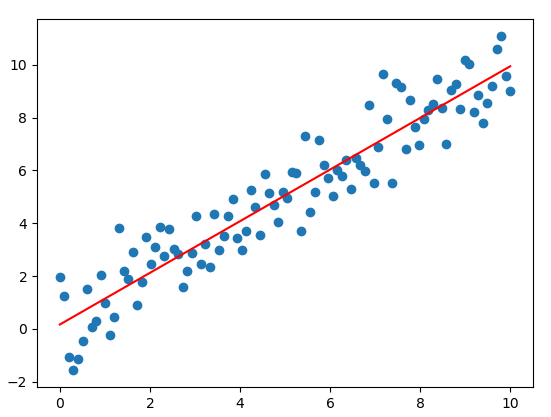{{announcement.body}}
{{announcement.title}}

# Learn TensorFlow: Creating the Linear Regression Model in TensorFlow 2

DZone 's Guide to

# Learn TensorFlow: Creating the Linear Regression Model in TensorFlow 2

### In this article, take a look at how to create the linear regression model in TensorFlow 2.

· AI Zone ·
Free Resource

Comment (1)

Save
{{ articles.views | formatCount}} Views

### Introduction to TensorFlow 2 (TF 2)

From TensorFlow Guide, there are major changes in TF 2:

• API Cleanup: Removing redundant APIs such as  `tf.app` , ` tf.flags` ,  `tf.logging`
• Eager Execution: Executing eagerly like Python
• No more globals: Keeping track of your variables
• Functions, not sessions: Can decorate a Python function using  `tf.function()`  to mark it for JIT compilation so that TensorFlow runs it as a single graph.

### Building Linear Regression in TF 2

In one of my older articles, I introduced the linear regression algorithm and how to create a simple linear regression model using TensorFlow 1.X. In this post, I will rebuild that model using TensorFlow 2.x as follows:

Python

`xxxxxxxxxx`
1
37

1
`import tensorflow as tf`
2
`import numpy as np`
3
`import matplotlib.pyplot as plt`
4

5
`learning_rate = 0.01`
6

7
`# steps of looping through all your data to update the parameters`
8
`training_epochs = 100`
9

10
`# the training set`
11
`x_train = np.linspace(0, 10, 100)`
12
`y_train = x_train + np.random.normal(0,1,100)`
13
`w0 = tf.Variable(0.)`
14
`w1 = tf.Variable(0.)`
15

16
`def h(x):`
17
`   y = w1*x + w0`
18
`   return y`
19

20
`def squared_error(y_pred, y_true):`
21
`   return tf.reduce_mean(tf.square(y_pred - y_true))`
22

23
`# train model`
24
`for epoch in range(training_epochs):`
25
`    with tf.GradientTape() as tape:`
26
`        y_predicted = h(x_train)`
27
`        costF = squared_error(y_predicted, y_train)`
28
`    # get gradients`
29
`    gradients = tape.gradient(costF, [w1,w0])`
30
`    # compute and adjust weights`
31
`    w1.assign_sub(gradients*learning_rate)`
32
`    w0.assign_sub(gradients*learning_rate)`
33

34
`plt.scatter(x_train, y_train)`
35
`# plot the best fit line`
36
`plt.plot(x_train, h(x_train), 'r')`
37
`plt.show()`

The result will look like this:We note some changes:

• declare variables ( `tf.Variable` ) but don't need to use  `tf.global_variables_initializer` , that means, TensorFlow 2.0 doesn’t make it mandatory to initialize variables.
• train our model using t `f.GradientTape ` and we will use  `assign_sub`  for weight variables.
• not require the session execution.

## Conclusion

TensorFlow is a great platform for deep learning and machine learning and TF 2.0 focuses on simplicity and ease of use. In this post, I introduced some new changes in TF 2.0 by building simple linear regression models from scratch (don't use APIs such as Keras), and I hope you feel excited about it.

Topics:
ai ,artificial intelligence ,machine learning ,open source ,python ,tensorflow 2.0 ,tutorial

Comment (1)

Save
{{ articles.views | formatCount}} Views

Opinions expressed by DZone contributors are their own.